# Checkmate

#### 2019-07-04

Ever used an R function that produced a not-very-helpful error message, just to discover after minutes of debugging that you simply passed a wrong argument?

Blaming the laziness of the package author for not doing such standard checks (in a dynamically typed language such as R) is at least partially unfair, as R makes theses types of checks cumbersome and annoying. Well, that’s how it was in the past.

Enter checkmate.

Virtually every standard type of user error when passing arguments into function can be caught with a simple, readable line which produces an informative error message in case. A substantial part of the package was written in C to minimize any worries about execution time overhead.

## Intro

As a motivational example, consider you have a function to calculate the faculty of a natural number and the user may choose between using either the stirling approximation or R’s factorial function (which internally uses the gamma function). Thus, you have two arguments, n and method. Argument n must obviously be a positive natural number and method must be either "stirling" or "factorial". Here is a version of all the hoops you need to jump through to ensure that these simple requirements are met:

fact <- function(n, method = "stirling") {
if (length(n) != 1)
stop("Argument 'n' must have length 1")
if (!is.numeric(n))
stop("Argument 'n' must be numeric")
if (is.na(n))
stop("Argument 'n' may not be NA")
if (is.double(n)) {
if (is.nan(n))
stop("Argument 'n' may not be NaN")
if (is.infinite(n))
stop("Argument 'n' must be finite")
if (abs(n - round(n, 0)) > sqrt(.Machine$double.eps)) stop("Argument 'n' must be an integerish value") n <- as.integer(n) } if (n < 0) stop("Argument 'n' must be >= 0") if (length(method) != 1) stop("Argument 'method' must have length 1") if (!is.character(method) || !method %in% c("stirling", "factorial")) stop("Argument 'method' must be either 'stirling' or 'factorial'") if (method == "factorial") factorial(n) else sqrt(2 * pi * n) * (n / exp(1))^n } And for comparison, here is the same function using checkmate: fact <- function(n, method = "stirling") { assertCount(n) assertChoice(method, c("stirling", "factorial")) if (method == "factorial") factorial(n) else sqrt(2 * pi * n) * (n / exp(1))^n } ## Function overview The functions can be split into four functional groups, indicated by their prefix. If prefixed with assert, an error is thrown if the corresponding check fails. Otherwise, the checked object is returned invisibly. There are many different coding styles out there in the wild, but most R programmers stick to either camelBack or underscore_case. Therefore, checkmate offers all functions in both flavors: assert_count is just an alias for assertCount but allows you to retain your favorite style. The family of functions prefixed with test always return the check result as logical value. Again, you can use test_count and testCount interchangeably. Functions starting with check return the error message as a string (or TRUE otherwise) and can be used if you need more control and, e.g., want to grep on the returned error message. expect is the last family of functions and is intended to be used with the testthat package. All performed checks are logged into the testthat reporter. Because testthat uses the underscore_case, the extension functions only come in the underscore style. All functions are categorized into objects to check on the package help page. ## In case you miss flexibility You can use assert to perform multiple checks at once and throw an assertion if all checks fail. Here is an example where we check that x is either of class foo or class bar: f <- function(x) { assert( checkClass(x, "foo"), checkClass(x, "bar") ) } Note that assert(, combine = "or") and assert(, combine = "and") allow to control the logical combination of the specified checks, and that the former is the default. ## Argument Checks for the Lazy The following functions allow a special syntax to define argument checks using a special format specification. E.g., qassert(x, "I+") asserts that x is an integer vector with at least one element and no missing values. This very simple domain specific language covers a large variety of frequent argument checks with only a few keystrokes. You choose what you like best. ## checkmate as testthat extension To extend testthat, you need to IMPORT, DEPEND or SUGGEST on the checkmate package. Here is a minimal example: # file: tests/test-all.R library(testthat) library(checkmate) # for testthat extensions test_check("mypkg") Now you are all set and can use more than 30 new expectations in your tests. test_that("checkmate is a sweet extension for testthat", { x = runif(100) expect_numeric(x, len = 100, any.missing = FALSE, lower = 0, upper = 1) # or, equivalent, using the lazy style: qexpect(x, "N100[0,1]") }) ## Speed considerations In comparison with tediously writing the checks yourself in R (c.f. factorial example at the beginning of the vignette), R is sometimes a tad faster while performing checks on scalars. This seems odd at first, because checkmate is mostly written in C and should be comparably fast. Yet many of the functions in the base package are not regular functions, but primitives. While primitives jump directly into the C code, checkmate has to use the considerably slower .Call interface. As a result, it is possible to write (very simple) checks using only the base functions which, under some circumstances, slightly outperform checkmate. However, if you go one step further and wrap the custom check into a function to convenient re-use it, the performance gain is often lost (see benchmark 1). For larger objects the tide has turned because checkmate avoids many unnecessary intermediate variables. Also note that the quick/lazy implementation in qassert/qtest/qexpect is often a tad faster because only two arguments have to be evaluated (the object and the rule) to determine the set of checks to perform. Below you find some (probably unrepresentative) benchmark. But also note that this one here has been executed from inside knitr which is often the cause for outliers in the measured execution time. Better run the benchmark yourself to get unbiased results. ### Benchmark 1: Assert that x is a flag library(checkmate) library(ggplot2) library(microbenchmark) x = TRUE r = function(x, na.ok = FALSE) { stopifnot(is.logical(x), length(x) == 1, na.ok || !is.na(x)) } cm = function(x) assertFlag(x) cmq = function(x) qassert(x, "B1") mb = microbenchmark(r(x), cm(x), cmq(x)) print(mb) ## Unit: microseconds ## expr min lq mean median uq max neval cld ## r(x) 2.417 2.6155 23.72241 2.7435 2.8350 2068.835 100 a ## cm(x) 1.650 1.7860 8.42189 1.8605 1.9495 573.437 100 a ## cmq(x) 1.141 1.2375 8.20890 1.3185 1.4100 614.834 100 a autoplot(mb) ## Coordinate system already present. Adding new coordinate system, which will replace the existing one.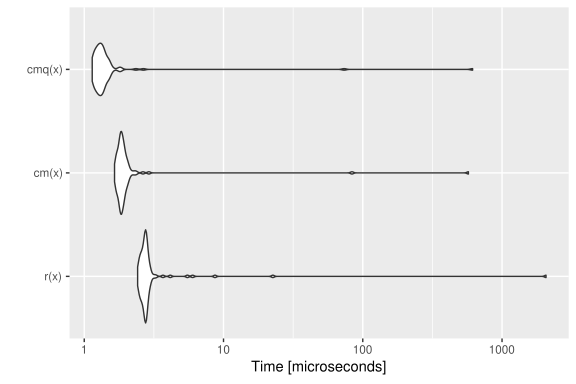### Benchmark 2: Assert that x is a numeric of length 1000 with no missing nor NaN values x = runif(1000) r = function(x) stopifnot(is.numeric(x), length(x) == 1000, all(!is.na(x) & x >= 0 & x <= 1)) cm = function(x) assertNumeric(x, len = 1000, any.missing = FALSE, lower = 0, upper = 1) cmq = function(x) qassert(x, "N1000[0,1]") mb = microbenchmark(r(x), cm(x), cmq(x)) print(mb) ## Unit: microseconds ## expr min lq mean median uq max neval cld ## r(x) 11.261 11.9935 38.88152 12.2730 12.8715 2608.472 100 a ## cm(x) 4.792 5.0115 12.79137 5.2410 5.5400 691.840 100 a ## cmq(x) 5.068 5.2665 13.42892 5.4655 5.7200 777.905 100 a autoplot(mb) ## Coordinate system already present. Adding new coordinate system, which will replace the existing one.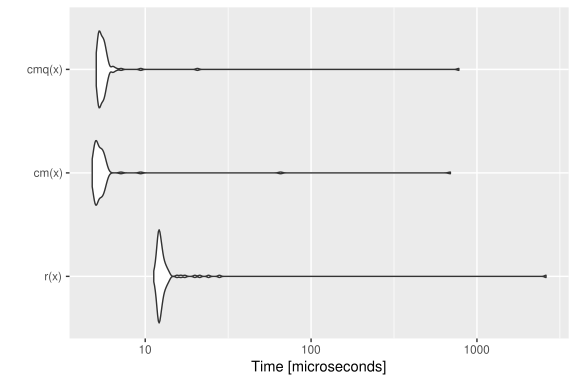### Benchmark 3: Assert that x is a character vector with no missing values nor empty strings x = sample(letters, 10000, replace = TRUE) r = function(x) stopifnot(is.character(x), !any(is.na(x)), all(nchar(x) > 0)) cm = function(x) assertCharacter(x, any.missing = FALSE, min.chars = 1) cmq = function(x) qassert(x, "S+[1,]") mb = microbenchmark(r(x), cm(x), cmq(x)) print(mb) ## Unit: microseconds ## expr min lq mean median uq max neval ## r(x) 1318.497 1320.4630 1355.09132 1322.979 1353.9675 3216.189 100 ## cm(x) 80.904 82.1415 91.73229 83.417 84.2290 742.297 100 ## cmq(x) 104.815 109.4095 120.48241 112.869 113.4235 898.126 100 ## cld ## b ## a ## a autoplot(mb) ## Coordinate system already present. Adding new coordinate system, which will replace the existing one.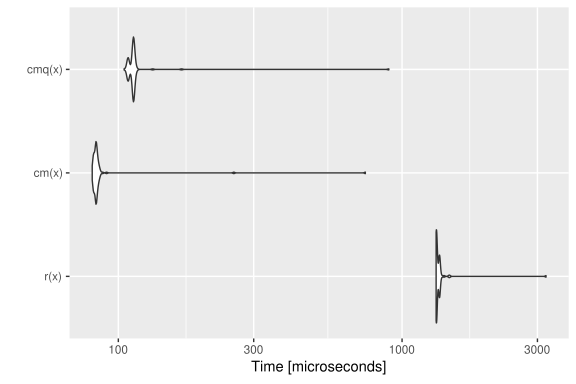### Benchmark 4: Test that x is a data frame with no missing values N = 10000 x = data.frame(a = runif(N), b = sample(letters[1:5], N, replace = TRUE), c = sample(c(FALSE, TRUE), N, replace = TRUE)) r = function(x) is.data.frame(x) && !any(sapply(x, function(x) any(is.na(x)))) cm = function(x) testDataFrame(x, any.missing = FALSE) cmq = function(x) qtest(x, "D") mb = microbenchmark(r(x), cm(x), cmq(x)) print(mb) ## Unit: microseconds ## expr min lq mean median uq max neval cld ## r(x) 71.713 73.5955 116.00141 76.2900 89.3430 3114.106 100 b ## cm(x) 16.883 17.6805 30.87342 18.8310 24.1300 725.735 100 a ## cmq(x) 10.672 11.0550 25.22804 11.3955 12.2195 1036.290 100 a autoplot(mb) ## Coordinate system already present. Adding new coordinate system, which will replace the existing one.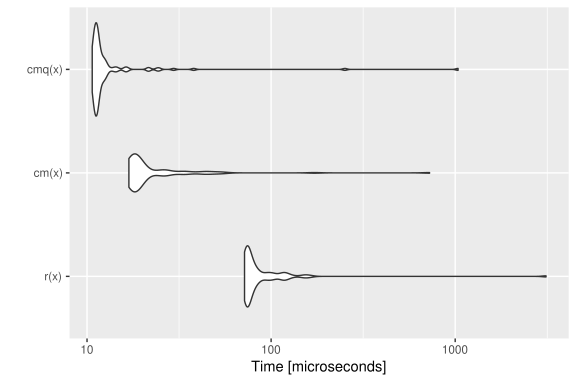# checkmate tries to stop as early as possible x$a = NA
mb = microbenchmark(r(x), cm(x), cmq(x))
print(mb)
## Unit: nanoseconds
##    expr   min      lq     mean  median      uq    max neval cld
##    r(x) 63372 64136.5 65969.08 64522.0 65825.0 112633   100   c
##   cm(x)  4171  4514.0  5409.27  5259.0  5728.0  18928   100  b
##  cmq(x)   810  1022.5  1416.16  1333.5  1622.5  10269   100 a
autoplot(mb)
## Coordinate system already present. Adding new coordinate system, which will replace the existing one.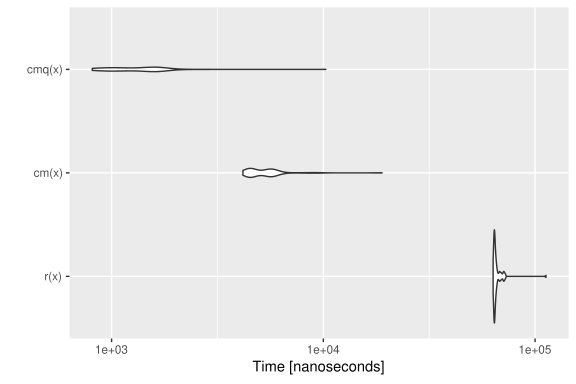### Benchmark 5: Assert that x is an increasing sequence of integers with no missing values

N = 10000
x.altrep = seq_len(N) # this is an ALTREP in R version >= 3.5.0
x.sexp = c(x.altrep)  # this is a regular SEXP OTOH
r = function(x) stopifnot(is.integer(x), !any(is.na(x)), !is.unsorted(x))
cm = function(x) assertInteger(x, any.missing = FALSE, sorted = TRUE)
mb = microbenchmark(r(x.sexp), cm(x.sexp), r(x.altrep), cm(x.altrep))
print(mb)
## Unit: microseconds
##          expr    min      lq     mean  median      uq      max neval cld
##     r(x.sexp) 27.178 27.5760 45.48629 27.8250 28.1535 1733.298   100   a
##    cm(x.sexp) 10.895 11.1235 11.50690 11.4085 11.7660   13.331   100   a
##   r(x.altrep) 30.737 30.9875 31.59164 31.2475 31.5660   36.900   100   a
##  cm(x.altrep)  2.605  3.0035 12.68487  3.4740  3.6460  838.409   100   a
autoplot(mb)
## Coordinate system already present. Adding new coordinate system, which will replace the existing one.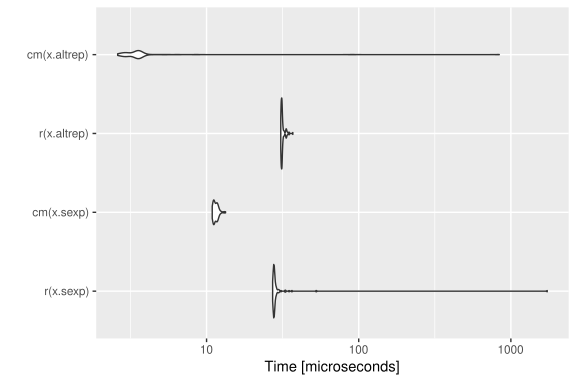## Extending checkmate

To extend checkmate a custom check* function has to be written. For example, to check for a square matrix one can re-use parts of checkmate and extend the check with additional functionality:

checkSquareMatrix = function(x, mode = NULL) {
# check functions must return TRUE on success
# and a custom error message otherwise
res = checkMatrix(x, mode = mode)
if (!isTRUE(res))
return(res)
if (nrow(x) != ncol(x))
return("Must be square")
return(TRUE)
}

# a quick test:
X = matrix(1:9, nrow = 3)
checkSquareMatrix(X)
##  TRUE
checkSquareMatrix(X, mode = "character")
##  "Must store characters"
checkSquareMatrix(X[1:2, ])
##  "Must be square"

The respective counterparts to the check-function can be created using the constructors makeAssertionFunction, makeTestFunction and makeExpectationFunction:

# For assertions:
assert_square_matrix = assertSquareMatrix = makeAssertionFunction(checkSquareMatrix)
print(assertSquareMatrix)
## function (x, mode = NULL, .var.name = checkmate::vname(x), add = NULL)
## {
##     if (missing(x))
##         stop(sprintf("argument \"%s\" is missing, with no default",
##             .var.name))
##     res = checkSquareMatrix(x, mode)
## }
# For tests:
test_square_matrix = testSquareMatrix = makeTestFunction(checkSquareMatrix)
print(testSquareMatrix)
## function (x, mode = NULL)
## {
##     isTRUE(checkSquareMatrix(x, mode))
## }
# For expectations:
expect_square_matrix = makeExpectationFunction(checkSquareMatrix)
print(expect_square_matrix)
## function (x, mode = NULL, info = NULL, label = vname(x))
## {
##     if (missing(x))
##         stop(sprintf("Argument '%s' is missing", label))
##     res = checkSquareMatrix(x, mode)
##     makeExpectation(x, res, info, label)
## }

Note that all the additional arguments .var.name, add, info and label are automatically joined with the function arguments of your custom check function. Also note that if you define these functions inside an R package, the constructors are called at build-time (thus, there is no negative impact on the runtime).

## Calling checkmate from C/C++

The package registers two functions which can be used in other packages’ C/C++ code for argument checks.

SEXP qassert(SEXP x, const char *rule, const char *name);
Rboolean qtest(SEXP x, const char *rule);

These are the counterparts to qassert and qtest. Due to their simplistic interface, they perfectly suit the requirements of most type checks in C/C++.

For detailed background information on the register mechanism, see the Exporting C Code section in Hadley’s Book “R Packages” or WRE. Here is a step-by-step guide to get you started:

1. Add checkmate to your “Imports” and “LinkingTo” sections in your DESCRIPTION file.
2. Create a stub C source file "checkmate_stub.c", see below.
3. Include the provided header file <checkmate.h> in each compilation unit where you want to use checkmate.

File contents for (2):

#include <checkmate.h>
#include <checkmate_stub.c>

## Session Info

For the sake of completeness, here the sessionInfo() for the benchmark (but remember the note before on knitr possibly biasing the results).

sessionInfo()
## R version 3.6.0 (2019-04-26)
## Platform: x86_64-pc-linux-gnu (64-bit)
## Running under: Arch Linux
##
## Matrix products: default
## BLAS/LAPACK: /usr/lib/libopenblas_haswellp-r0.3.6.so
##
## locale:
##   LC_CTYPE=de_DE.UTF-8       LC_NUMERIC=C
##   LC_TIME=de_DE.UTF-8        LC_COLLATE=C
##   LC_MONETARY=de_DE.UTF-8    LC_MESSAGES=de_DE.UTF-8
##   LC_PAPER=de_DE.UTF-8       LC_NAME=C
##  LC_MEASUREMENT=de_DE.UTF-8 LC_IDENTIFICATION=C
##
## attached base packages:
##  stats     graphics  grDevices utils     datasets  methods   base
##
## other attached packages:
##  microbenchmark_1.4-6 ggplot2_3.2.0        checkmate_1.9.4
##
## loaded via a namespace (and not attached):
##   Rcpp_1.0.1        pillar_1.4.2      compiler_3.6.0
##   tools_3.6.0       digest_0.6.19     evaluate_0.14
##   tibble_2.1.3      gtable_0.3.0      lattice_0.20-38
##  pkgconfig_2.0.2   rlang_0.4.0       Matrix_1.2-17
##  yaml_2.2.0        mvtnorm_1.0-11    xfun_0.8
##  withr_2.1.2       stringr_1.4.0     dplyr_0.8.2
##  knitr_1.23        grid_3.6.0        tidyselect_0.2.5
##  glue_1.3.1        R6_2.4.0          survival_2.44-1.1
##  rmarkdown_1.13    multcomp_1.4-10   TH.data_1.0-10
##  purrr_0.3.2       magrittr_1.5      codetools_0.2-16
##  MASS_7.3-51.4     splines_3.6.0     backports_1.1.4
##  scales_1.0.0      htmltools_0.3.6   assertthat_0.2.1
##  colorspace_1.4-1  sandwich_2.5-1    stringi_1.4.3
##  lazyeval_0.2.2    munsell_0.5.0     crayon_1.3.4
##  zoo_1.8-6